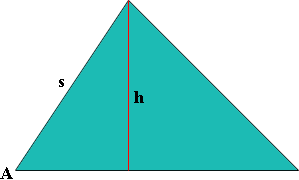SEARCH HOMEMath Central Quandaries & QueriesQuestion from saba, a student: the three sides of a triangular lot have lengths 10,11and 13cm,respectively. find the measure of its largest angle and the area of the lot?Hi Saba,

Which angle has the largest measure? If that is not clear from the numbers then draw a reasonably accurate diagram. Use the Law of Cosines to calculate the measure of the angle.

You can use Heron's Formula to calculate the area of the triangle directly from the lengths of the three sides. If you want to use the fact that the area of a triangle is "half the base times the height" then consider the diagramand the fact that $\sin A = \large \frac{h}{s}.$

PennyMath Central is supported by the University of Regina and The Pacific Institute for the Mathematical Sciences.